# AQA A Level Maths: Statistics复习笔记4.2.2 Calculating Binomial Probabilities

### Calculating Binomial Probabilities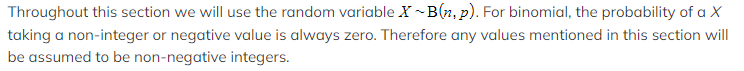#### How do I calculate, P(X = x) the probability of a single value for a binomial distribution?

• You should have a calculator that can calculate binomial probabilities
• You want to use the "Binomial Probability Distribution" function
• This is sometimes shortened to BPD, Binomial PD or Binomial Pdf
• You will need to enter: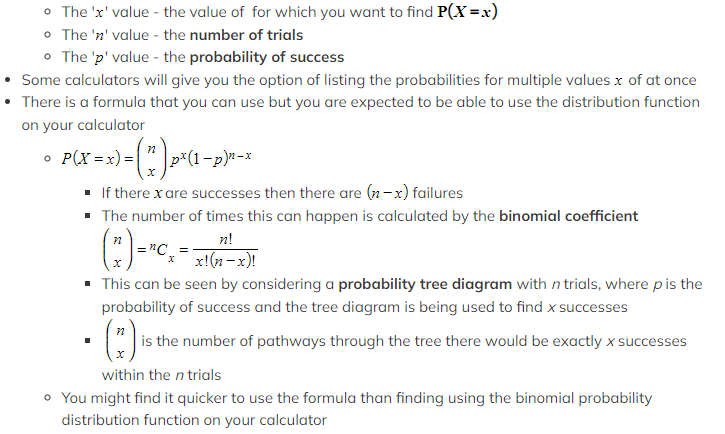#### How do I calculate, P(X ≤ x) , the cumulative probabilities for a binomial distribution?

• You should have a calculator that can calculate cumulative binomial probabilities

####How do I find P(X ≥ x)?

• You might be lucky enough to have a calculator that has lower and upper bounds:

####How do I find  P(a ≤ X ≤ b) ?

• You might be lucky enough to have a calculator that has lower and upper bounds:
• Use a for the lower bound and b for the upper bound
• Otherwise, you will need some extra identities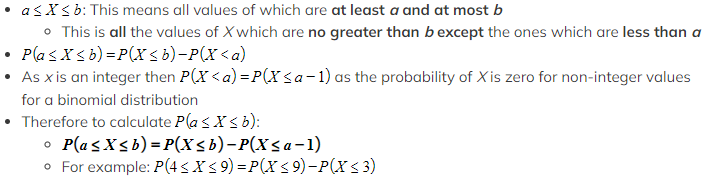####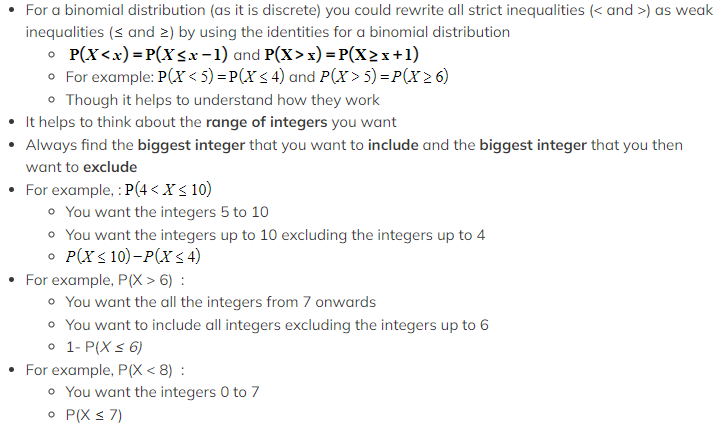Worked Example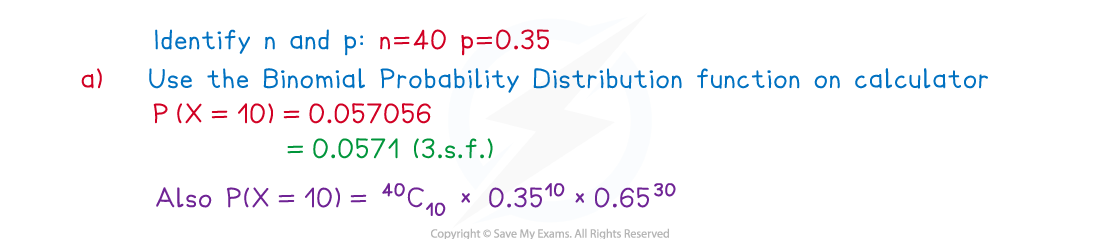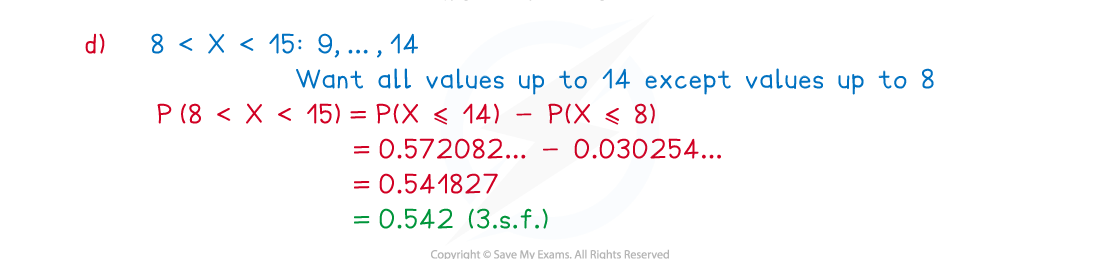#### Exam Tip

• Always make sure you are using the correct function on your calculator. Most questions will be in context so try and pick out the key words and numbers. If the question is worth more than one mark then be sure to show a method to get at least one mark if you write the answer down incorrectly.